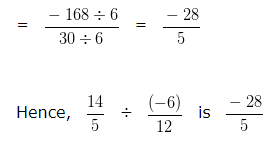# Dividing Rational Numbers

In order to divide a Rational Number by another Rational Number
We have to multiply first Rational Number with Reciprocal of the second Rational Number.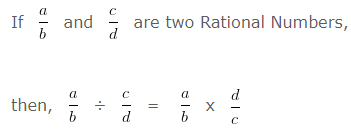### Dividing Rational Numbers Examples

Example 1

Divide:

9/7 ÷ 3/4

Explanation

We have, 9/7 ÷ 3/4

In order to divide a Rational Number by another Rational Number

We have to multiply first Rational Number with Reciprocal of the second Rational Number.

We have 9/7 ÷ 3/4

(Reciprocal of 3/4 is 4/3 )

So we can say that,

9/7 ÷ 3/4

= 9/7 x 4/3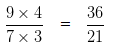To further simplify the given numbers into their lowest form, we would divide both the Numerator and Denominator by their HCF

HCF of 36 and 21 is 3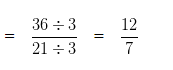Dividing Rational Numbers Example 2

Divide:

14/5 ÷ -6/12

Explanation

We have, 14/5 ÷ 6/12

In order to divide a Rational Number by another Rational Number

We have to multiply first Rational Number with Reciprocal of the second Rational Number

Since, Reciprocal of -6/12 is 12/-6

We can write the given equation as 14/5 ÷ -6/12

= 14/5 x 12/-6

To make the Denominator positive, we would multiply 12 and -6 by -1

Given equation would now be 14/5 x -12/6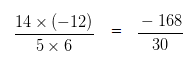To further simplify the given numbers into their lowest form, we would divide both the Numerator and Denominator by their HCF

HCF of 168 and 30 is 6